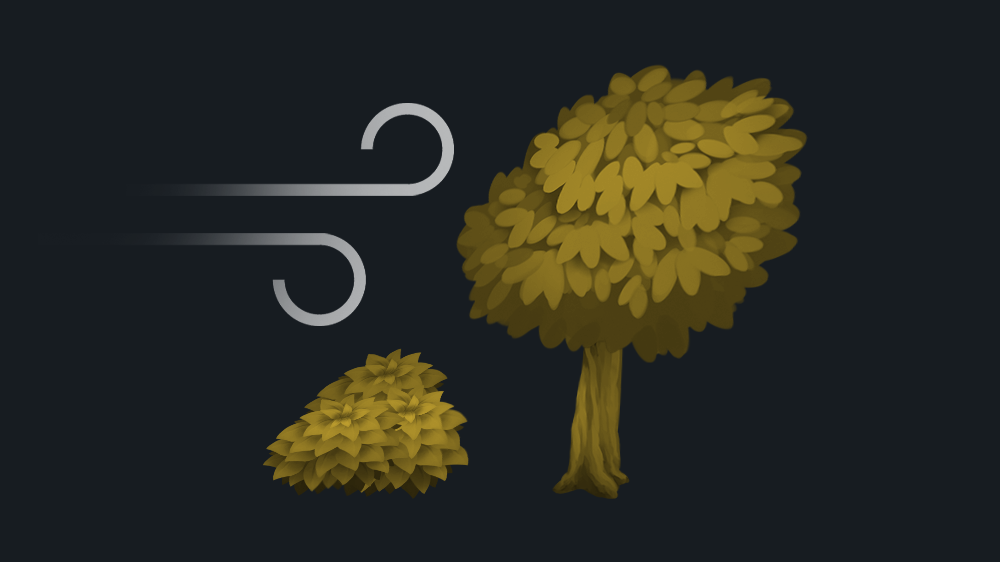# 2D wind sway

Make a sprite sway, like it is affected by the wind. See the animation below for example. There are several parameters to control:

• Speed – The speed of the wind movement.
• Min Strength – The minimal strength of the wind movement.
• Max Strength – The maximal strength of the wind movement.
• Strength Scale – Scalefactor for the wind strength.
• Interval – The time between minimal and maximal strength changes.
• Detail – The detail (number of waves) of the wind movement.
• Distortion – The strength of geometry distortion.
• Height Offset – The height where the wind begins to move. By default 0.0.
• Offset – Set different moves for each asset.
``````// original wind shader from https://github.com/Maujoe/godot-simple-wind-shader-2d/tree/master/assets/maujoe.simple_wind_shader_2d
// original script modified by HungryProton so that the assets are moving differently : https://pastebin.com/VL3AfV8D
//
// speed - The speed of the wind movement.
// minStrength - The minimal strength of the wind movement.
// maxStrength - The maximal strength of the wind movement.
// strengthScale - Scalefactor for the wind strength.
// interval - The time between minimal and maximal strength changes.
// detail - The detail (number of waves) of the wind movement.
// distortion - The strength of geometry distortion.
// heightOffset - The height where the wind begins to move. By default 0.0.

render_mode blend_mix;

// Wind settings.
uniform float speed = 1.0;
uniform float minStrength : hint_range(0.0, 1.0) = 0.05;
uniform float maxStrength : hint_range(0.0, 1.0) = 0.01;
uniform float strengthScale = 100.0;
uniform float interval = 3.5;
uniform float detail = 1.0;
uniform float distortion : hint_range(0.0, 1.0);
uniform float heightOffset : hint_range(0.0, 1.0);

// With the offset value, you can if you want different moves for each asset. Just put a random value (1, 2, 3) in the editor. Don't forget to mark the material as unique if you use this
uniform float offset = 0;

float getWind(vec2 vertex, vec2 uv, float time){
float diff = pow(maxStrength - minStrength, 2.0);
float strength = clamp(minStrength + diff + sin(time / interval) * diff, minStrength, maxStrength) * strengthScale;
float wind = (sin(time) + cos(time * detail)) * strength * max(0.0, (1.0-uv.y) - heightOffset);

return wind;
}

void vertex() {
vec4 pos = WORLD_MATRIX * vec4(0.0, 0.0, 0.0, 1.0);
float time = TIME * speed + offset;
//float time = TIME * speed + pos.x * pos.y  ; not working when moving...
VERTEX.x += getWind(VERTEX.xy, UV, time);
}``````
###### Tags
2d, tree, vertex, windThe shader code and all code snippets in this post are under CC0 license and can be used freely without the author's permission. Images and videos, and assets depicted in those, do not fall under this license. For more info, see our License terms.

## More from godotshaders

### Wind Waker 2d Water Shader Canvas_ItemInline Feedbacksmohd
1 year ago

how do you use the shadersummer
1 year ago